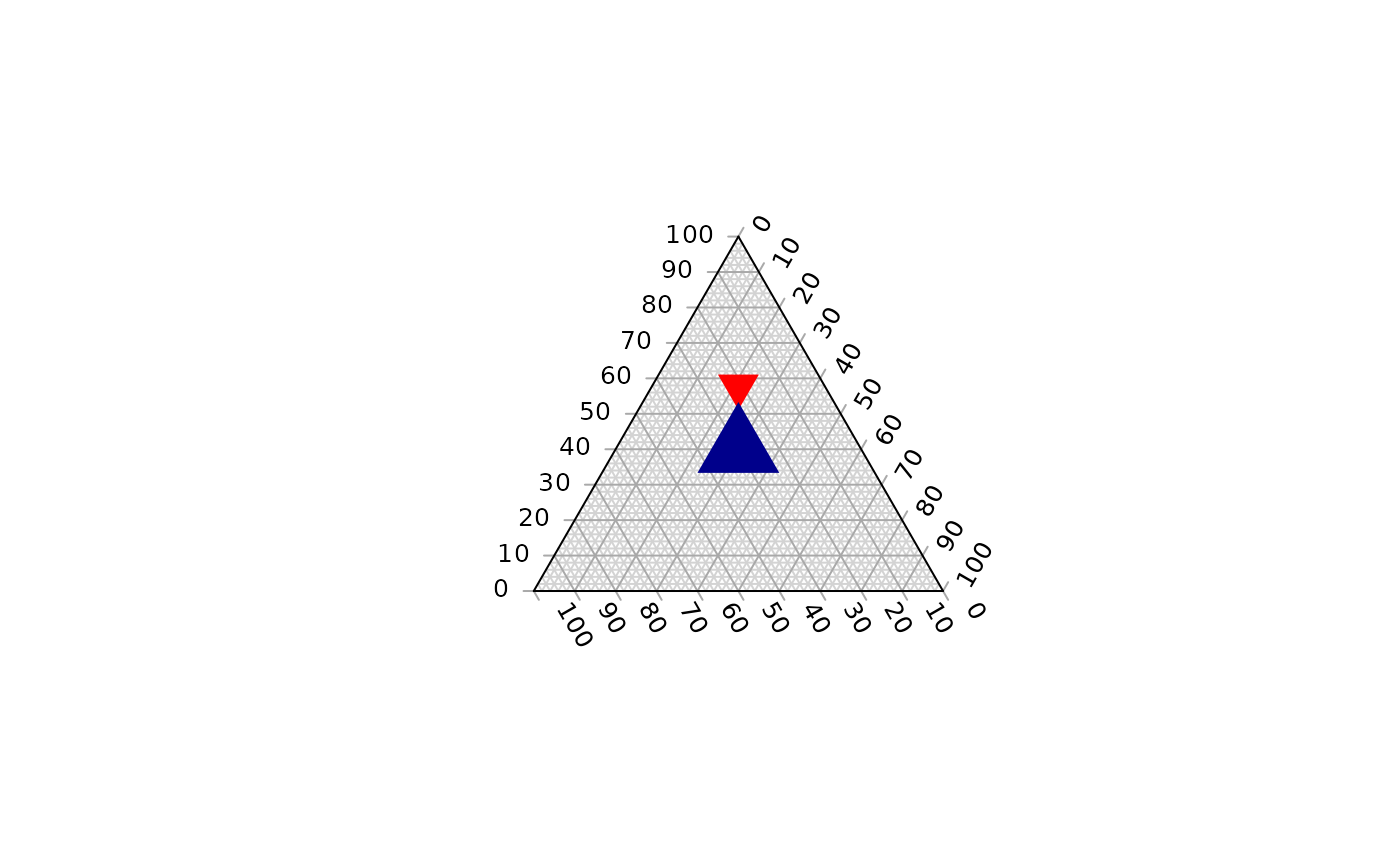Function to fill a ternary plot with coloured tiles. Useful in combination with TernaryPointValues and TernaryContour.

TernaryTiles(
x,
y,
down,
resolution,
col,
direction = getOption("ternDirection", 1L)
)

## Arguments

x, y

Numeric vectors specifying x and y coordinates of centres of each triangle.

down

Logical vector specifying TRUE if each triangle should point down (or right), FALSE otherwise.

resolution

The number of triangles whose base should lie on the longest axis of the triangle. Higher numbers will result in smaller subdivisions and smoother colour gradients, but at a computational cost.

col

Vector specifying the colour with which to fill each triangle.

direction

(optional) Integer specifying the direction that the current ternary plot should point: 1, up; 2, right; 3, down; 4, left.

Other functions for colouring and shading: ColourTernary()

## Examples


FunctionToContour <- function (a, b, c) {
a - c + (4 * a * b) + (27 * a * b * c)
}

TernaryPlot()

values <- TernaryPointValues(FunctionToContour, resolution = 24L)
ColourTernary(values)
TernaryContour(FunctionToContour, resolution=36L)